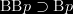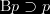If one believes that they believe that p, then do they believe that p ($\text{B} \text{B} p \supset \text{B} p$)?
I was recently thinking about this property and its absence from standard systems of doxastic logic. Systems of doxastic logic rightly do not validate the property$\text{B} p \supset p$. Since they omit this axiom (commonly called the T axiom),$\text{B} \text{B} p \supset \text{B} p$ cannot be simply derived. But although the T axiom should not be valid in a doxastic logic, it is fair to say that the axiom$\text{B} \text{B} p \supset \text{B} p$ should be valid; if one believes that they believe that p, then they do believe that p.